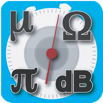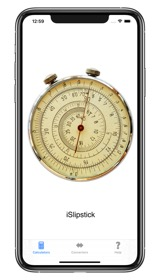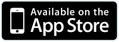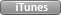iSlipstick is a collection of commonly used electronics formula calculators and converters.iSlipstick includes the following calculators:

OHM’S LAW - Voltage, Current, Resistance and Power.

OP-AMP GAIN - Find the gain of an inverting or non-inverting op-amp.

REACTANCE - Find inductive or capacitive reactance.

RESONANCE - Find the resonance of an LC circuit.

VOLTAGE DIVIDER - Find the output voltage of a two resistor series circuit.

PARALLEL RESISTOR - Find the sum resistance of up to 6 resistors in parallel.

SERIES RLC - Find the impedance and phase angle of a series RLC circuit.

PARALLEL RLC - Find the impedance and phase angle of a parallel RLC circuit.

iSlipstick also includes:

VOLTAGE CONVERSION - RMS, average, peak and peak-to-peak.

RESISTOR COLOR CODE - Find the color code of 4 and 5 band resistors and vice-versa.

SMT RESISTOR - 3 digit, 4 digit and EIA-96 code decoder.

dB CONVERTER - dBm (50, 75 and 600 ohm), dBW, dBV and dBuV.

TEMPERATURE CONVERSION - Convert between Fahrenheit, Celsius, Kelvin and Rankine.

PERIOD to FREQUENCY - Convert period to frequency and vice-versa.

LENGTH, AREA, VOLUME, CAPACITY and WEIGHT - Convert between metric and US imperial and within each standard.

Purchase iSlipstick on the iTunes App Store.``